Electron. J. Differential Equations, Vol. 2018 (2018), No. 105, pp. 1-24.

### Existence of solutions to nonlinear p-Laplacian fractional differential equations with higher-order derivative terms You-Hui Su, Yongzhen Yun, Dongdong Wang, Weimin Hu

Abstract:
In this article, we discuss the existence of positive solution to a nonlinear p-Laplacian fractional differential equation whose nonlinearity contains a higher-order derivative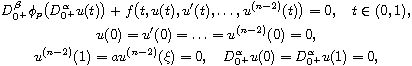where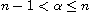,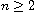,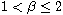,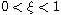,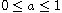and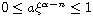,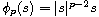,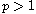,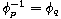,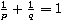.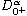,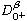are the standard Riemann-Liouville fractional derivatives, and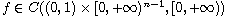. The Green's function of the fractional differential equation mentioned above and its relevant properties are presented, and some novel results on the existence of positive solution are established by using the mixed monotone fixed point theorem and the upper and lower solution method. The interesting of this paper is that the nonlinearity involves the higher-order derivative, and also, two examples are given in this paper to illustrate our main results from the perspective of application.

Submitted June 19, 2016. Published May 7, 2018.
Math Subject Classifications: 34B25, 34B18, 35G30.
Key Words: Fractional differential equation; Green's function; p-Laplacian operator; upper and lower solution method.

Show me the PDF file (315 KB), TEX file for this article.You-Hui Su School of Mathematics and Physics Xuzhou University of Technology Xuzhou, Jiangsu 221018, China email: suyh02@163.com, suyouhui@xzit.edu.cn Yongzhen Yun College of Science Hohai University Nanjing, Jiangsu 211100, China email: yongzhen0614@163.com Dongdong Wang School of Mathematics and Physics Xuzhou University of Technology Xuzhou, Jiangsu 221018, China email: wdd@xzit.edu.cn Weimin Hu School of Mathematics and Statistic Yili Normal University Yining, Xinjiang 835000, China email: hwm680702@163.com